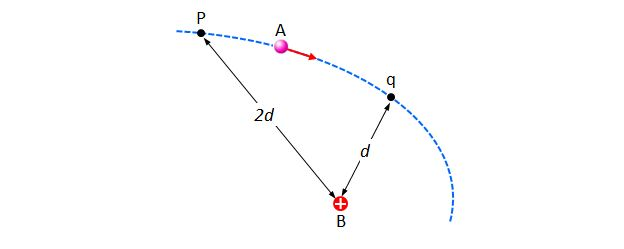# Moving point chargeThe above diagram shows the moving path of a point charge A which receives the electric force from a fixed point charge $B$ which is positively charged. The distances between B and the two points p and q are $2d$ and $d,$ respectively. Which of the following statements is correct?

a) The point charge A is negatively charged.
b) The electric potential exerted by the point charge B at the point p is higher than that at the point q.
c) The magnitude of the electrostatic force acting on the point charge B at the point p is greater than that at the point q.

×